# Machine Learning and Generalization Error — Is Learning Possible?

Machine learning is about building models based on some given sample data, also known as training data, and afterward using this model to make predictions and decisions on new, unknown data. Hence, it can be said that machine learning is about learning and using the rules intrinsic to the training data. This where a problem arises — how can we be sure whether the rules the model has learned from the training data are viable on new, unseen data? This article will be the first in a series of articles concerning the theory of generalization in machine learning. In this article, the learning problem will be clearly defined.

Definition of Generalization Error

Since this series is concerned with understanding generalization error and how to minimize it, it is important to first define what it is. Simply described, generalization error is a measure of how well a machine learning model performs (i.e., predicts) on previously, unseen data. Hence, the smaller it is — the better. This is also called the out-of-sample error, which will be further explained later in this article. With this out of the way, let us get started.

Learning the Rules

Let us imagine that we have a dataset, D, which consists of multiple samples — each sample consists of some observations and its outcome. This our training data, and we want our model to learn to predict based on that. To make this possible, we need to do is to find a function, f, which we will call the target function. Intuitively the target function can be compared to the well-known mathematical function, f(x) — the function decides the output based upon the input. Easy, is it not? Well, one problem — the target function is unknown to us and always will be!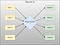An illustration of how the target function decides the output based on the input. This is the case inside our dataset, D, but also outside it. The target function is unknown.

Since the target function is unknown, except inside our sample, how can we then know whether our approximated function is the good one? Any function that agrees with the target function inside D could possibly be the correct one! Each of these possible functions is called a hypothesis, and the set of possible functions is called the hypothesis class, H.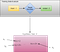A very simple example of a hypothesis class. We have a training set, in this case with only one sample. When we give input, 1, we seem to get the output, 2 — from that, we need to guess a good function to estimate the target function. Our hypothesis class consists of all possible functions that give this result — only 3 are shown here, but there are up to n of these functions. The question is then to choose the good one!

If our target function, f, is unknown then we cannot exclude any values of ‘f’ outside of D, our given training set. What we can do, though, is to use probability to infer something about outside D using only D. This will be explained intuitively through examples. If you do not know anything about Hoeffding’s Inequality, then it is advised to first read my article about it, since it will be highly relevant in the following section.

Hoeffding Saves the Day

We will now see how probability will be able to help us estimate our target function f, simply by using our sample dataset, D. We start with a simple example.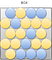A closed box containing blue and yellow balls. We CANNOT look inside this box.

We consider a box — it contains blue and yellow balls inside it. The important point is that we cannot look inside the box — it is closed to our eyes. What we can do, though, is to perform experiments on it. To do so, we will first need to define some things — we need to define the probability of picking either a blue or a yellow ball.

Remember, µ is simply a variable name since we do not know the actual probability of picking a yellow ball! Now comes the interesting part — we can reach into our box and take a sample. We pick N balls independently. We then observe the fraction of yellow balls in our sample, this fraction will be called v.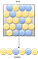We take a random, independent sample from our box!

The million-dollar question is then — can this sample tell us anything about our unknown distribution in the box? Absolutely! If the sample is big enough — then v will probably be close to µ! We can describe it with the following, familiar equation: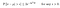Hoeffding’s Inequality

In other words, the equation says that as the sample size, N, grows, it becomes exponentially unlikely that v will deviate from µ by more than our ‘tolerance’, ε. Notice that only the size N of the sample affects the bound, not the size of the ‘box’. The ‘box’ can be large or small, finite or infinite, and we still get the same bound when we use the same sample size. If we choose ε to be small to make v a good approximation of µ, we need a larger sample size N. The thing worth noting is: µ does not impact our probability bound! We will now see why exactly this is interesting.

From Box to Learning

In our box example, µ was the unknown — in our learning situation, it is the target function, f. We can use the box as a direct example — it might get a bit technical and we will need to understand an important definition: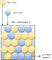We relate the box to our learning problem — here our box is the input space with each ball being a point. The yellow points are where a hypothesis function agrees with the target function.

The yellow balls are the points that our h(x) is getting right according to the target function, f(x). So, if h(x) agrees with f(x), then the ball is colored yellow. If the balls are colored blue, then our hypothesis disagrees with the target function. Now there is a probability associated with the box — The color that each point gets is not known to us since f is unknown. However, if we pick x at random according to some probability distribution P over the input space X, we know that x will be yellow with some probability, call it µ, and blue with probability 1 — µ.

Regardless of the value of µ, the space X now behaves like the example with the box. The learning problem is now reduced to a box problem, under the assumption that the inputs in D are picked independently according to some distribution, P, on X, our input space. Any P will translate to some µ in the equivalent box. Since µ can be unknown, then P can as well. We will now need to change some of the notation.

This can be directly translated to Hoeffding’s inequality — instead of approximating the difference between mu and v, we are trying to approximate the difference between E_in and E_out.The Hoeffding’s Inequality with E_in and E_out substituted in.

Learning or Verification?

Now the question is the following: is this learning or verification? It seems more like verification than actual learning since we simply check the viability of a single, chosen hypothesis rather than searching for the optimal one. Luckily, this can be solved.

We simply need to apply the idea above to multiple boxes instead of a single one — We can say that each box is a hypothesis for our target function, and we want to find a good one — before we had one single box and we had to check whether it seemed likely with our sample from the target function. This time we can have many boxes and we need to verify all of them to check which one seems most likely. This time, we generalize to a finite number of boxes.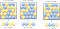We generalize to a finite amount of boxes!

We have one problem though — Hoeffding’s Inequality doesn’t apply to multiple boxes! We have an assumption with Hoeffding’s Inequality that the hypothesis function is fixed before the data set. With multiple hypotheses, i.e. considering a whole hypothesis set, the learning algorithm picks the final hypothesis, g, based on D. That means it’s picked after the dataset is generated. How can this be solved?

Slacking the Bound

The way to get around this is to try to bound P|E_in(g) — E_out(g)| > ε| in a way so it does not depend on a specific, chosen hypothesis. The way to do this is to take the union of all the probability bounds on all the hypotheses in the hypothesis set, H.

We then get the following inequality:

The downside is that the inequality is a factor of M looser than the bound for a single hypothesis, and will only be meaningful if M, our number of hypotheses in H, is finite. But do not despair — we will see in the following articles that we can improve upon this bound!

Conclusion

The conclusion is that we can see that, yes, learning is feasible! By adopting a probabilistic view, it makes it possible to conclude that learning is possible. Not even a particular probability distribution is needed, or even on knowing which one is used. The only assumption needed is that the examples in our sample data are independently generated. We also only need to estimate how well our chosen hypothesis, g, corresponds to the target function, f. This is the reason that Hoeffding’s Inequality is such a great match.

References:

— — — — — — — — — — — — — — — — — — — — — — — — -

 H-S. Lin, M. Magdon-Ismail, Y. S. Abu-Mostafa, Learning From Data — a short course (2012).

 Y. Sin, Understanding Generalization Error in Machine Learning (2018), https://medium.com/@yixinsun_56102/understanding-generalization-error-in-machine-learning-e6c03b203036

Data science and Machine Learning student at Copenhagen University.

## More from Naja Møgeltoft

Data science and Machine Learning student at Copenhagen University.